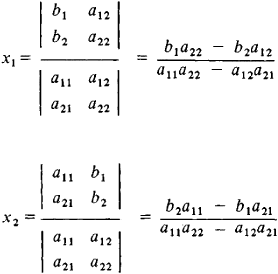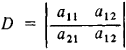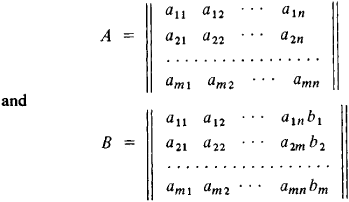Linear Equation

(redirected from Slope intercept form)
Also found in: Dictionary, Thesaurus, Medical.
Related to Slope intercept form: Standard form

linear equation

[′lin·ē·ər i′kwā·zhən]
(mathematics)
A linear equation in the variables x1, … , xn , and y is any equation of the form a1 x1+ a2 x2+ … + an xn = y.

Linear Equation

an equation in which the unknowns are of first degree (that is, linear) and which has no terms containing products of the unknowns. Several linear equations in the same unknowns form a system of linear equations. A solution of a system of linear equations is a set of numbers c1, c2,. . ., cn that convert all the equations of the system into identities when put in place of the appropriate unknowns. A system of linear equations may have a unique solution or an infinite number of solutions (an indeterminate system); sometimes the system of linear equations has no solutions (an inconsistent system).

The case in which the number of equations coincides with the number of unknowns is encountered most frequently. One linear equation in one unknown has the form ax = b. When a ≠ 0, the number b/a will be its solution. A system of two linear equations in two unknowns has the form

(1) a11x1 + a12x2 = b1

a12x1 + a22x2 = b2

where a11, a12, a21, a22, b1, and b2 are given numbers. The solution of system (1) can be obtained by using determinants:Here it is assumed that the determinantin the denominator differs from zero. The numerators are determinants obtained from D by replacing a column of D with the column of the free terms b1 and b2. the first column is replaced in the expression for the first unknown x1, and the second column is replaced in the expression for the second unknown x2.

An analogous rule is also applicable in solving any system of n linear equations in n unknowns, that is, a system of the type

a11x1 + a12x2 + ⋯ + a1nxn = b1

(2) a21x1 + a22x2 + ⋯ + a2nxn = b2

. . . . . . . . . . . . . . . . . . . . . . . . . . . . . . . . . .

an1x1 + an2x2 + ⋯ + annxn = bn

Here aij and bi (i, j = 1, 2,. . ., n) are arbitrary numerical coefficients; the numbers b1, b2, ..., bn are usually called free terms. If the determinant D = ǀaijǀ of system (2), which consists of the coefficients aij (the multipliers of the unknowns), differs from zero, then the solution is obtained in the following manner: the Kth (k = 1, 2,. . ., n) unknown xk is equal to a fraction whose denominator is the determinant D and whose numerator is the determinant obtained from D by replacing its kth column with the column of free terms b1, b2, . .., bn. If D = 0, then system (2) has either no solution or an infinite number of solutions.

If all bi = 0 (in this case the system of linear equations is said to be homogeneous) and D ≠ 0, then the only solution of system (2) will be the zero solution (that is, all xk = 0). In practice, we often encounter homogeneous systems of linear equations in which the number of equations is one less than the number of unknowns, that is, systems of the type

a11x1 + a12x2 + ⋯ + a1nxn = 0

(3) a21x1 + a22x2 + ⋯ + a2nxn = 0

. . . . . . . . . . . . . . . . . . . . . . . . . . . . . . . . . . . . .

an -1,1x1 + an - 1, 2x2 + ⋯ + an - 1, nxn = 0

The solution of such a system is not unique; generally, only the ratio of the unknowns can be found from it:

x1 : x2 : ⋯ : xn = D1 : D2 : ⋯ : Dn

where Dk is the product of (–1)k and the determinant obtained from the matrix of the coefficients aij of system (3) by crossing out its kth column (this rule is applicable only when at least one of the determinants Di, differs from zero).

The first solution of system (2) was given by G. Cramer in 1750; the rule for finding the solution of this system is still called Cramer’s rule. A complete theory of systems of linear equations was given 100 years later by L. Kronecker.

A general system of m linear equations in n unknowns has the form

a11x1 + a12x2 + ⋯ + a1nxn = b1

(4) a21x1 + a22x2 + ⋯ + a2nxn = b2

. . . . . . . . . . . . . . . . . . . . . . . . . . . . . . . . . . . . .

am1x1 + am2x2 + ⋯ + am nxn = bm

The question of the compatibility of the system of linear equations (4), that is, the question of whether a solution exists, is answered by comparing the ranks of the matricesIf the ranks are coincident, then the system is consistent; if the rank of matrix B is greater than the rank of matrix A, then the system is inconsistent (the Kronecker-Capelli theorem). If the system is consistent, its solution can be found in the following manner. After a nonzero minor of the highest order r is found in the matrix A, the mr equations whose coefficients were not part of this minor are dropped (the dropped equations will be consequences of the remaining ones and therefore need not be considered). In the remaining equations those unknowns whose coefficients are not part of the selected minor (the free unknowns) are moved to the right. After any numerical value is assigned to the free unknowns, a system of r equations in r unknowns that can be solved according to Cramer’s rule is obtained. The determined values of the r unknowns together with the values of the free unknowns will give a particular solution of system (4) (that is, one of many possible solutions). It is possible to express the other unknowns directly in terms of the free unknowns without assigning specific values to the latter. In this way we obtain a general solution, that is, a solution in which the unknowns are expressed in terms of parameters. By assigning arbitrary values to these parameters, it is possible to obtain all particular solutions of the system.

Homogeneous systems of linear equations can be solved in the same way. Their solutions have the property that the sum, difference, and, in general, any linear combination of solutions (considered as n-dimensional vectors) will also be a solution of the system. In other words, the set of solutions of a homogeneous system of linear equations forms a subspace of n-dimensional space. A set of linearly independent solutions whose linear combinations yield all solutions of a homogeneous system of linear equations (that is, a basis of the subspace of solutions) is called a fundamental system of solutions (of the homogeneous system of linear equations).

There is a simple relationship between the solutions of the system of linear equations (4) and the corresponding homogeneous system of linear equations (that is, equations with the same coefficients for the unknowns but with free terms equal to zero): the general solution of a nonhomogeneous system is obtained from the general solution of the corresponding homogeneous system by adding to it a particular solution of the nonhomogeneous system of linear equations.

The intuitive appeal of the theory of linear equations is enhanced by using the language of geometry. By introducing linear operators on vector spaces (by considering equations of the type Ax = b, where A is a linear operator and x and b are vectors), it is easy to establish the relation between algebraic linear equations and linear equations in infinite-dimensional spaces (systems of linear equations with an infinite number of unknowns) and, in particular, linear equations in function spaces, such as linear differential equations and linear integral equations.

The use of Cramer’s rule in the practical solution of systems of large numbers of linear equations may lead to considerable difficulties, since finding determinants of high order entails extremely cumbersome calculations. Therefore, various methods of numerical (approximate) solution of systems of linear equations have been developed.

REFERENCES

Entsiklopediia elementarnoi matematiki, book 2. Edited by P. S. Aleksandrov [et al.] Moscow-Leningrad, 1951.
Faddeev, D. K., and V. N. Faddeeva. Vychislitel’nye metody lineinoi algebry, 2nd ed. Moscow-Leningrad, 1963.
Site: Follow: Share:
Open / Close